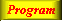Lecture 2 - Page 45 : 46
 Functional Programming in SchemeExpressions, Types, and Functions * Lisp and Scheme Lisp Scheme * Expressions and values Expressions, values, and types Examples of expressions and their values Evaluation of parenthesized expressions Arithmetic expressions Equality in Scheme The read-eval-print loop * Types Types Type checking Static type checking An example of type checking Types in functional programming languages * Lists Proper lists Symbolic expressions and improper lists Practical list construction List functions Association lists Property lists Tables as lists of rows Programs represented as lists * Other Data Types Other simple types Vectors Strings * Definitions Definitions * Functions The function concept Lambda calculus Functions in Scheme Function objects Functions as first class values Anonymous functions Lambda expressions in Scheme Optional parameters of Scheme functions (1) Optional parameters of Scheme functions (2) Closures Function definition in Scheme Simple web-related functions (1) Simple web-related functions (2) Function exercises
 Simple web-related functions (2) We will here show another simple function.
 ```(define (indent-pixels p . contents) (table 'border "0" (tbody (tr (td 'width (as-string p) "") (td 'width "*" contents)))))```

The definition of the indent-pixel function. This is a function which we use in many web documents to indent the contents a number of pixels relative to its context. Here we implement the indentation by use of a table, in which the first column cell is empty. As we will se, other possibilities exist.An alternative version of theindent-pixel function.

This version uses Cascading Style Sheets expressiveness. As it appears, this is a more compact, and more direct way of achieving our indentation goal.A sample application of indent-pixel with some initial LAML context (software loading).

Notice the use of the XHTML mirror.